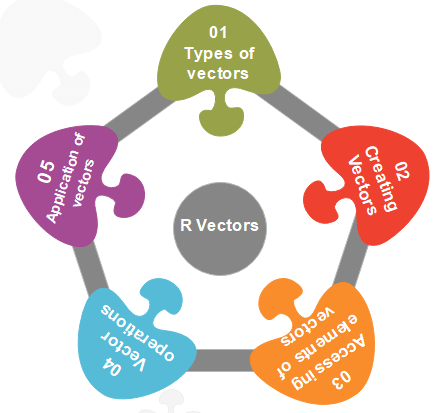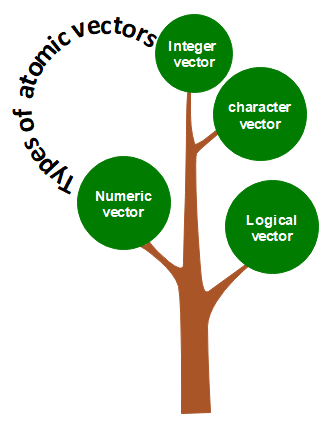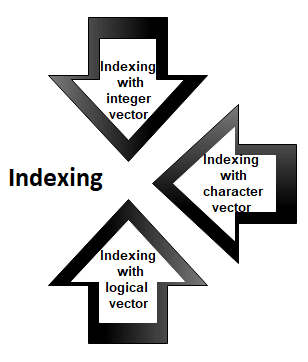# R Vector

A vector is a basic data structure which plays an important role in R programming.

In R, a sequence of elements which share the same data type is known as vector. A vector supports logical, integer, double, character, complex, or raw data type. The elements which are contained in vector known as components of the vector. We can check the type of vector with the help of the typeof() function.The length is an important property of a vector. A vector length is basically the number of elements in the vector, and it is calculated with the help of the length() function.

Vector is classified into two parts, i.e., Atomic vectors and Lists . They have three common properties, i.e., function type, function length , and attribute function .There is only one difference between atomic vectors and lists. In an atomic vector, all the elements are of the same type, but in the list, the elements are of different data types. In this section, we will discuss only the atomic vectors. We will discuss lists briefly in the next topic.

## How to create a vector in R?

In R, we use c() function to create a vector. This function returns a one-dimensional array or simply vector. The c() function is a generic function which combines its argument. All arguments are restricted with a common data type which is the type of the returned value. There are various other ways to create a vector in R, which are as follows:

### 1) Using the colon`(:)` operator

We can create a vector with the help of the colon operator. There is the following syntax to use colon operator:

z<-x:y

This operator creates a vector with elements from x to y and assigns it to z.

Example:

a<-4:-10
a

Output

 4 3 2 1 0 -1 -2 -3 -4 -5 -6 -7 -8 -9 -10

`

2) Using the seq() function
In R, we can create a vector with the help of the seq() function. A sequence function creates a sequence of elements as a vector. The seq() function is used in two ways, i.e., by setting step size with ?by’ parameter or specifying the length of the vector with the ‘length.out’ feature.

Example:

seq_vec<-seq(1,4,by=0.5)
seq_vec
class(seq_vec)

Output

 1.0 1.5 2.0 2.5 3.0 3.5 4.0

Example:

seq_vec<-seq(1,4,length.out=6)
seq_vec
class(seq_vec)

Output

 1.0 1.6 2.2 2.8 3.4 4.0
 “numeric”

## Atomic vectors in R

In R, there are four types of atomic vectors. Atomic vectors play an important role in Data Science. Atomic vectors are created with the help of c() function. These atomic vectors are as follows:### Numeric vector

The decimal values are known as numeric data types in R. If we assign a decimal value to any variable d, then this d variable will become a numeric type. A vector which contains numeric elements is known as a numeric vector.

Example:

d<-45.5
num_vec<-c(10.1, 10.2, 33.2)
d
num_vec
class(d)
class(num_vec)

Output

``````   45.5
   10.1   10.2   33.2
   "numeric"
   "numeric"
``````

### Integer vector

A non-fraction numeric value is known as integer data. This integer data is represented by “Int.” The Int size is 2 bytes and long Int size of 4 bytes. There is two way to assign an integer value to a variable, i.e., by using as.integer() function and appending of L to the value.

A vector which contains integer elements is known as an integer vector.

Example:

d<-as.integer(5)
e<-5L
int_vec<-c(1,2,3,4,5)
int_vec<-as.integer(int_vec)
int_vec1<-c(1L,2L,3L,4L,5L)
class(d)
class(e)
class(int_vec)
class(int_vec1)

Output

 “integer”
 “integer”
 “integer”
 “integer”

### Character vector

A character is held as a one-byte integer in memory. In R, there are two different ways to create a character data type value, i.e., using as.character() function and by typing string between double quotes("") or single quotes(’’).

A vector which contains character elements is known as an integer vector.

Example:

``````d<-'shubham'
e<-"Arpita"
f<-65
f<-as.character(f)
d
e
f
char_vec<-c(1,2,3,4,5)
char_vec<-as.character(char_vec)
char_vec1<-c("shubham","arpita","nishka","vaishali")
char_vec
class(d)
class(e)
class(f)
class(char_vec)
class(char_vec1)
``````

Output

 “shubham”
 “Arpita”
 “65”
 “1” “2” “3” “4” “5”
 “shubham” “arpita” “nishka” “vaishali”
 “character”
 “character”
 “character”
 “character”
 “character”

### Logical vector

The logical data types have only two values i.e., True or False. These values are based on which condition is satisfied. A vector which contains Boolean values is known as the logical vector.

Example:

d<-as.integer(5)
e<-as.integer(6)
f<-as.integer(7)
g<-d>e
h<-e<f
g
h
log_vec<-c(d<e, d<f, e<d,e<f,f<d,f<e)
log_vec
class(g)
class(h)
class(log_vec)

Output

 FALSE
 TRUE
 TRUE TRUE FALSE TRUE FALSE FALSE
 “logical”
 “logical”
 “logical”

## Accessing elements of vectors

We can access the elements of a vector with the help of vector indexing. Indexing denotes the position where the value in a vector is stored. Indexing will be performed with the help of integer, character, or logic.### 1) Indexing with integer vector

On integer vector, indexing is performed in the same way as we have applied in C, C++, and java. There is only one difference, i.e., in C, C++, and java the indexing starts from 0, but in R, the indexing starts from 1. Like other programming languages, we perform indexing by specifying an integer value in square braces [] next to our vector.

Example:

seq_vec<-seq(1,4,length.out=6)
seq_vec
seq_vec

Output

 1.0 1.6 2.2 2.8 3.4 4.0
 1.6

### 2) Indexing with a character vector

In character vector indexing, we assign a unique key to each element of the vector. These keys are uniquely defined as each element and can be accessed very easily. Let’s see an example to understand how it is performed.

Example:

char_vec<-c(“shubham”=22,“arpita”=23,“vaishali”=25)
char_vec
char_vec[“arpita”]

Output

shubham arpita vaishali
22 23 25
arpita
23

### 3) Indexing with a logical vector

In logical indexing, it returns the values of those positions whose corresponding position has a logical vector TRUE. Let see an example to understand how it is performed on vectors.

Example:

``````a<-c(1,2,3,4,5,6)
a[c(TRUE,FALSE,TRUE,TRUE,FALSE,TRUE)]
``````

Output

 1 3 4 6